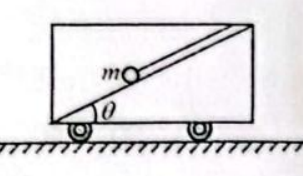$\text{A.}$ $1:3$ $\text{B.}$ $\sqrt{3}:3$ $\text{C.}$ $3:1$ $\text{D.}$ $\sqrt{3}:1$

A
①点击 收藏 此题， 扫码注册关注公众号接收信息推送（一月四份试卷,中1+高2+研1）
② 程序开发、服务器资源都需要大量的钱，如果你感觉本站好或者受到到帮助，欢迎赞助本站,赞助方式：微信/支付宝转账到 18155261033

 ①此题难易度如何 ②此题推荐度如何 确定